Exercises

Published

September 5, 2022

library(bayesrules)
library(tidyverse)
library(brms)
library(tidybayes)

# Plot stuff
clrs <- MetBrewer::met.brewer("Lakota", 6)
theme_set(theme_bw())

# Seed stuff
BAYES_SEED <- 1234
set.seed(1234)

## 4.9.2

The local ice cream shop is open until it runs out of ice cream for the day. It’s 2 p.m. and Chad wants to pick up an ice cream cone. He asks his coworkers about the chance (π) that the shop is still open. Their Beta priors for π are below:

worker_priors <- tribble(
~coworker, ~prior,
"Kimya", "Beta(1, 2)",
"Fernando", "Beta(0.5, 1)",
"Ciara", "Beta(3, 10)",
"Taylor", "Beta(2, 0.1)"
)

worker_priors %>% knitr::kable()
coworker prior
Kimya Beta(1, 2)
Fernando Beta(0.5, 1)
Ciara Beta(3, 10)
Taylor Beta(2, 0.1)

### 4.4: Choice of prior

Visualize and summarize (in words) each coworker’s prior understanding of Chad’s chances to satisfy his ice cream craving.

worker_prior_densities <- worker_priors %>%
mutate(shapes = str_match_all(prior, "Beta\$$(\\d+\\.?\\d*), (\\d+\\.?\\d*)\$$")) %>%
mutate(shape1 = map_dbl(shapes, ~as.numeric(.x)),
shape2 = map_dbl(shapes, ~as.numeric(.x))) %>%
mutate(range = list(seq(0, 1, length.out = 1001))) %>%
mutate(density = pmap(list(range, shape1, shape2), ~dbeta(..1, ..2, ..3)))

worker_prior_densities %>%
unnest(c(range, density)) %>%
# Truncate this a little for plotting
filter(range <= 0.99, range >= 0.01) %>%
ggplot(aes(x = range, y = density, fill = coworker, color = coworker)) +
geom_line(size = 1) +
geom_area(position = position_identity(), alpha = 0.4) +
scale_fill_manual(values = clrs[c(1, 2, 3, 5)]) +
scale_color_manual(values = clrs[c(1, 2, 3, 5)])
## Warning: Using size aesthetic for lines was deprecated in ggplot2 3.4.0.
## ℹ Please use linewidth instead.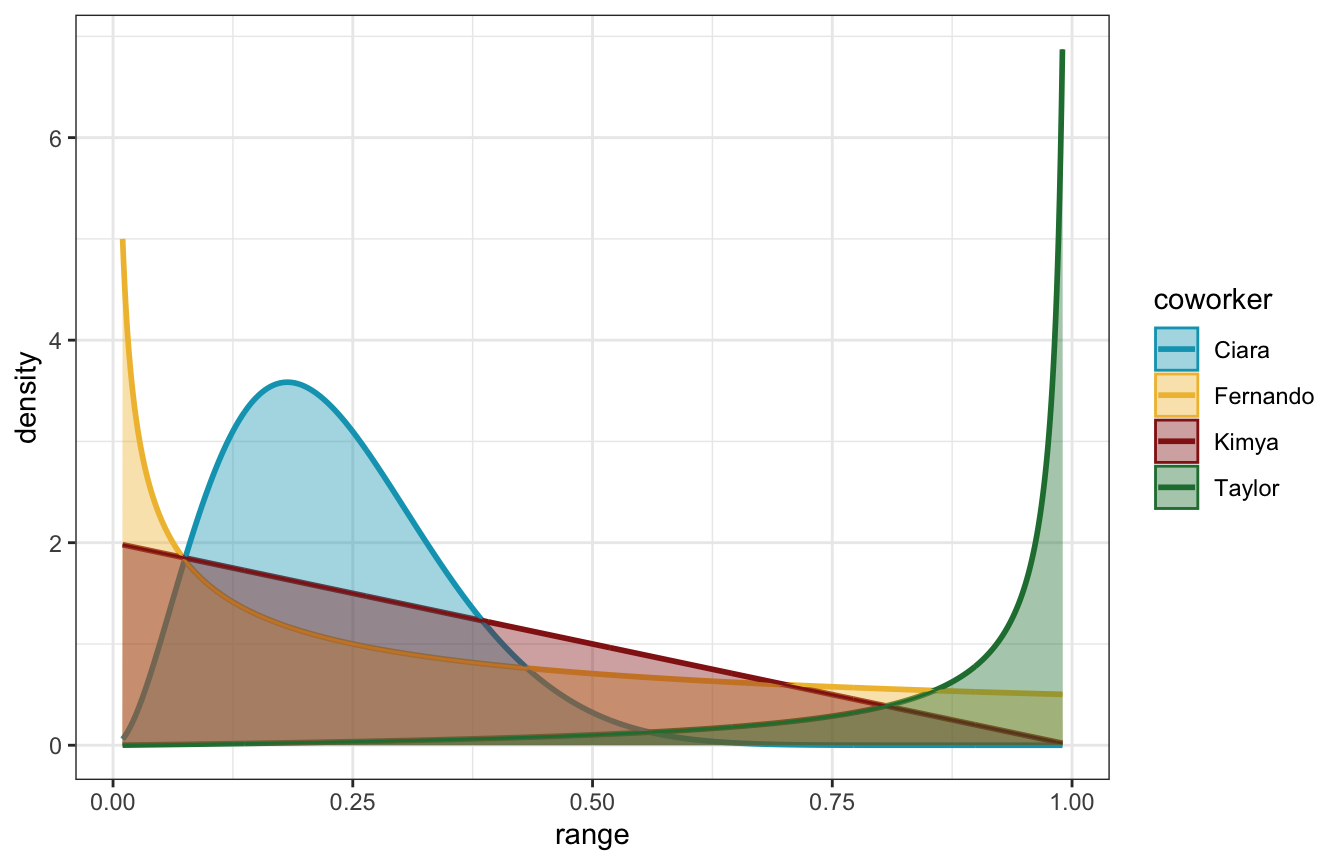• Kimya is relatively uncertain, but leaning towards believing that π is low
• Fernando puts heavy weight on very low probabilities of π—moreso than Kimya
• Ciara believes that π is clustered around 20%, and no higher than 50%
• Taylor is the most optimistic, believing that π is exceptionally high (like 90+%)

### 4.5 & 4.6: Simulating and identifying the posterior

Chad peruses the shop’s website. On 3 of the past 7 days, they were still open at 2 p.m.. Complete the following for each of Chad’s coworkers:

• simulate their posterior model;
• create a histogram for the simulated posterior; and
• use the simulation to approximate the posterior mean value of π

and

Complete the following for each of Chad’s coworkers:

• identify the exact posterior model of π;
• calculate the exact posterior mean of π; and
• compare these to the simulation results in the previous exercise.

#### Ciara only

\begin{aligned} (Y = 3) \mid \pi &= \operatorname{Binomial}(7, \pi) \\ \pi &\sim \operatorname{Beta}(3, 10) \end{aligned}

The Bayes Rules simulation approach is to simulate all the possibly likelihoods (here 0–7 / 7) and then filter to just choose one (3)

pi_ciara <- tibble(pi = rbeta(10000, 3, 10)) %>%
mutate(y = rbinom(10000, size = 7, prob = pi))

ggplot(pi_ciara, aes(x = pi, y = y)) +
geom_point(aes(color = (y == 3)))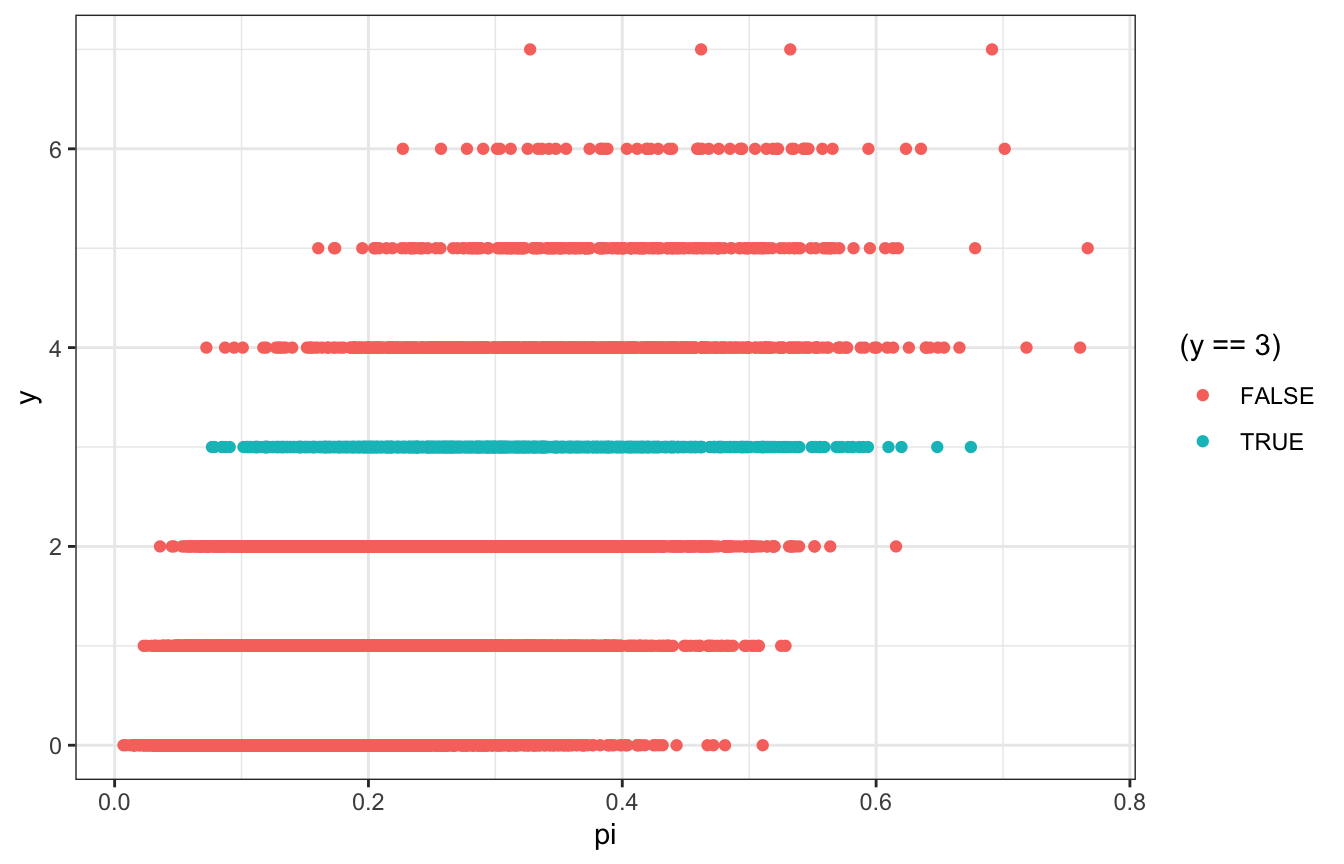pi_ciara %>%
filter(y == 3) %>%
summarize(across(pi, lst(mean, median)))
## # A tibble: 1 × 2
##   pi_mean pi_median
##     <dbl>     <dbl>
## 1   0.302     0.296

pi_ciara %>%
filter(y == 3) %>%
ggplot(aes(x = pi)) +
geom_density()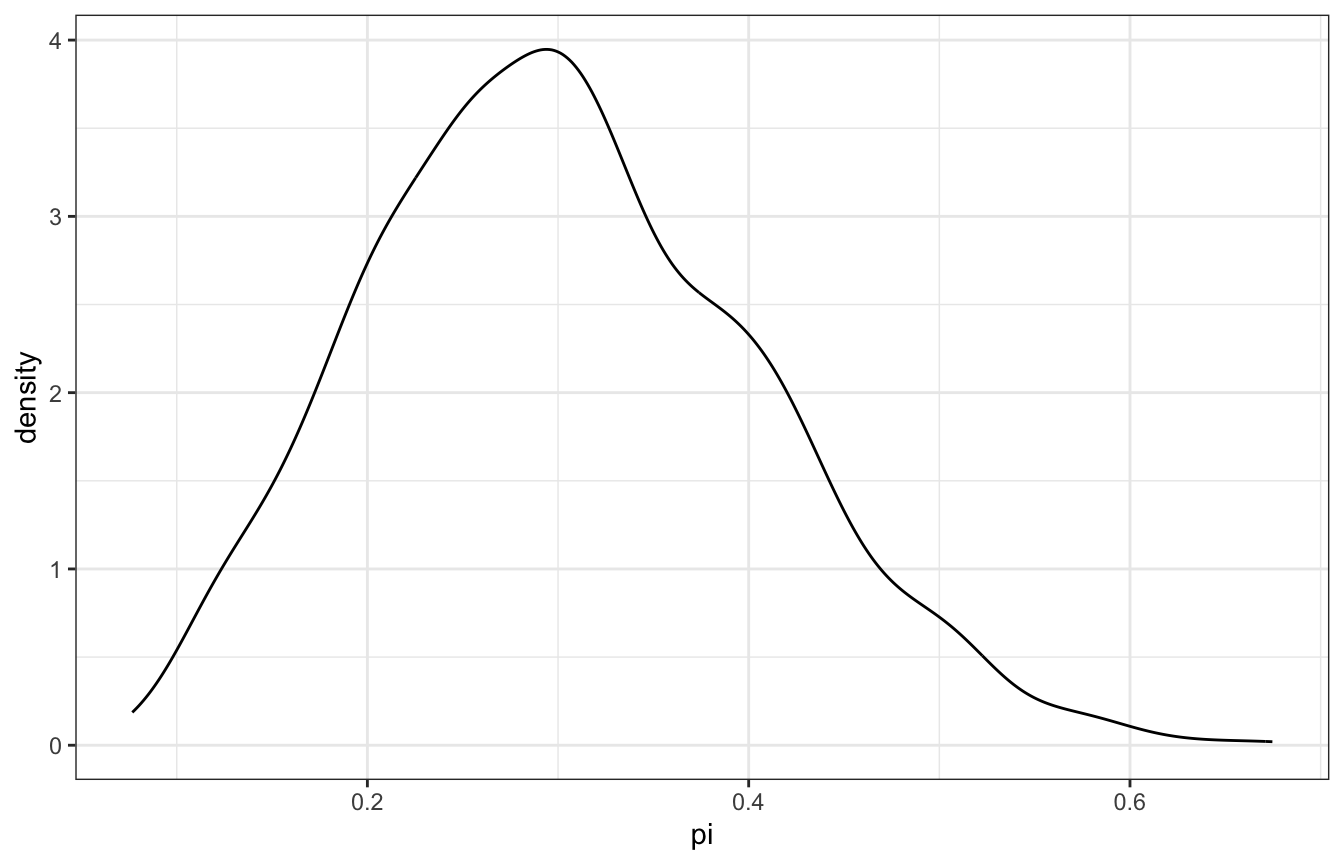pi_grid <- tibble(pi_grid = seq(0, 1, length.out = 1001)) %>%
mutate(prior_beta = dbeta(pi_grid, 3, 10)) %>%
mutate(likelihood = dbinom(3, size = 7, prob = pi_grid)) %>%
mutate(posterior = (likelihood * prior_beta) / sum(likelihood * prior_beta))

pi_samples <- pi_grid %>%
slice_sample(n = 10000, weight_by = posterior, replace = TRUE)

pi_samples %>%
summarize(across(pi_grid, lst(mean, median)))
## # A tibble: 1 × 2
##   pi_grid_mean pi_grid_median
##          <dbl>          <dbl>
## 1        0.301          0.295

ggplot(pi_samples, aes(x = pi_grid)) +
geom_density()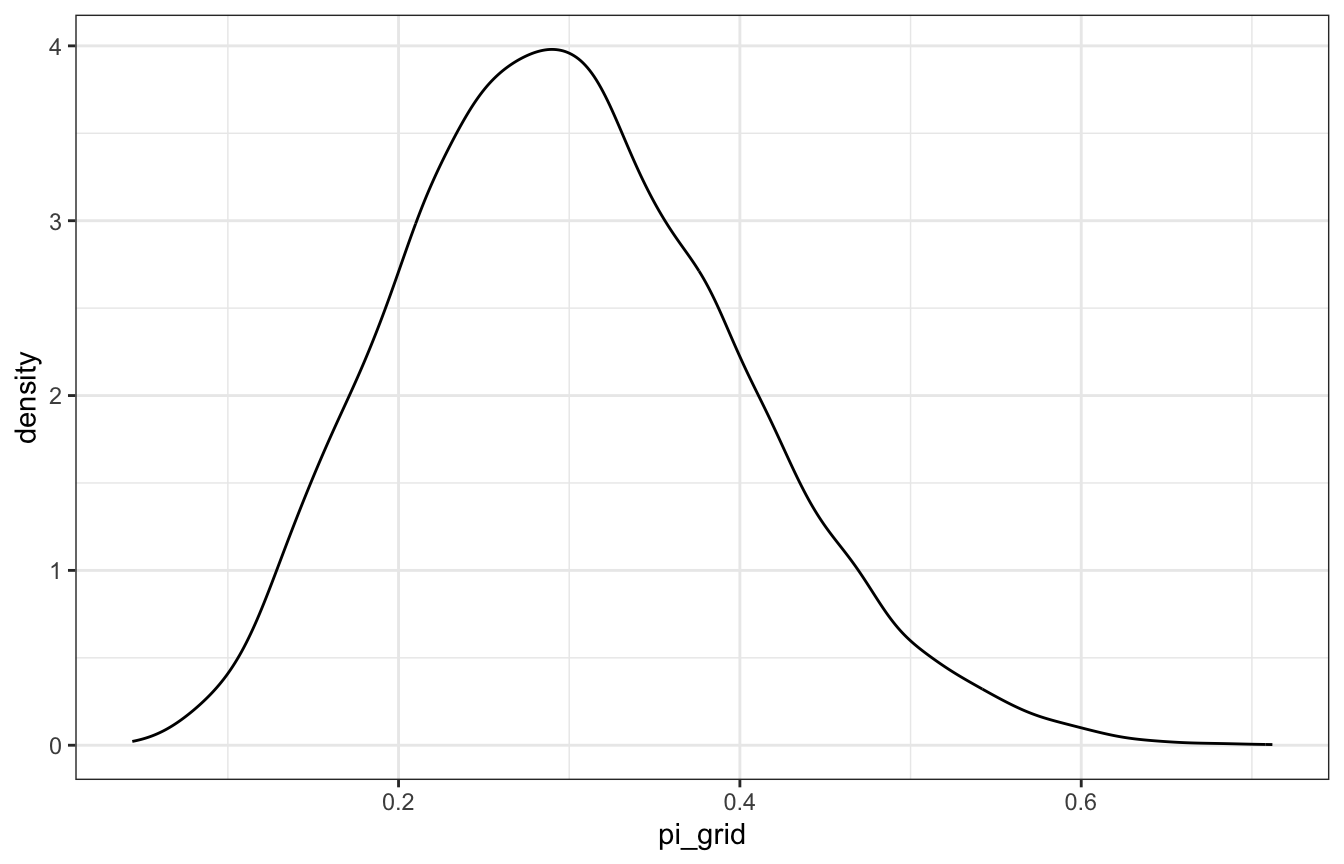model_pi <- brm(
bf(days_open | trials(weekdays) ~ 0 + Intercept),
data = list(days_open = 3, weekdays = 7),
prior(beta(3, 10), class = b, lb = 0, ub = 1),
iter = 5000, warmup = 1000, seed = BAYES_SEED,
backend = "rstan", cores = 4
)
## Compiling Stan program...
## Start sampling
model_pi %>%
summarize(across(b_Intercept, lst(mean, median, hdci = ~median_hdci(., width = 0.89)))) %>%
unnest(b_Intercept_hdci)
## # A tibble: 1 × 8
##   b_Intercept_mean b_Intercept_median     y  ymin  ymax .width .point .interval
##              <dbl>              <dbl> <dbl> <dbl> <dbl>  <dbl> <chr>  <chr>
## 1            0.298              0.292 0.292 0.110 0.493   0.95 median hdci
model_pi %>%
gather_draws(b_Intercept) %>%
ggplot(aes(x = .value)) +
geom_density(aes(fill = "Posterior"), color = NA, alpha = 0.75) +
stat_function(geom = "area", fun = ~dbeta(., 3, 10), aes(fill = "Beta(3, 10) prior"), alpha = 0.75) +
scale_fill_manual(values = clrs[5:6]) +
xlim(c(0, 1))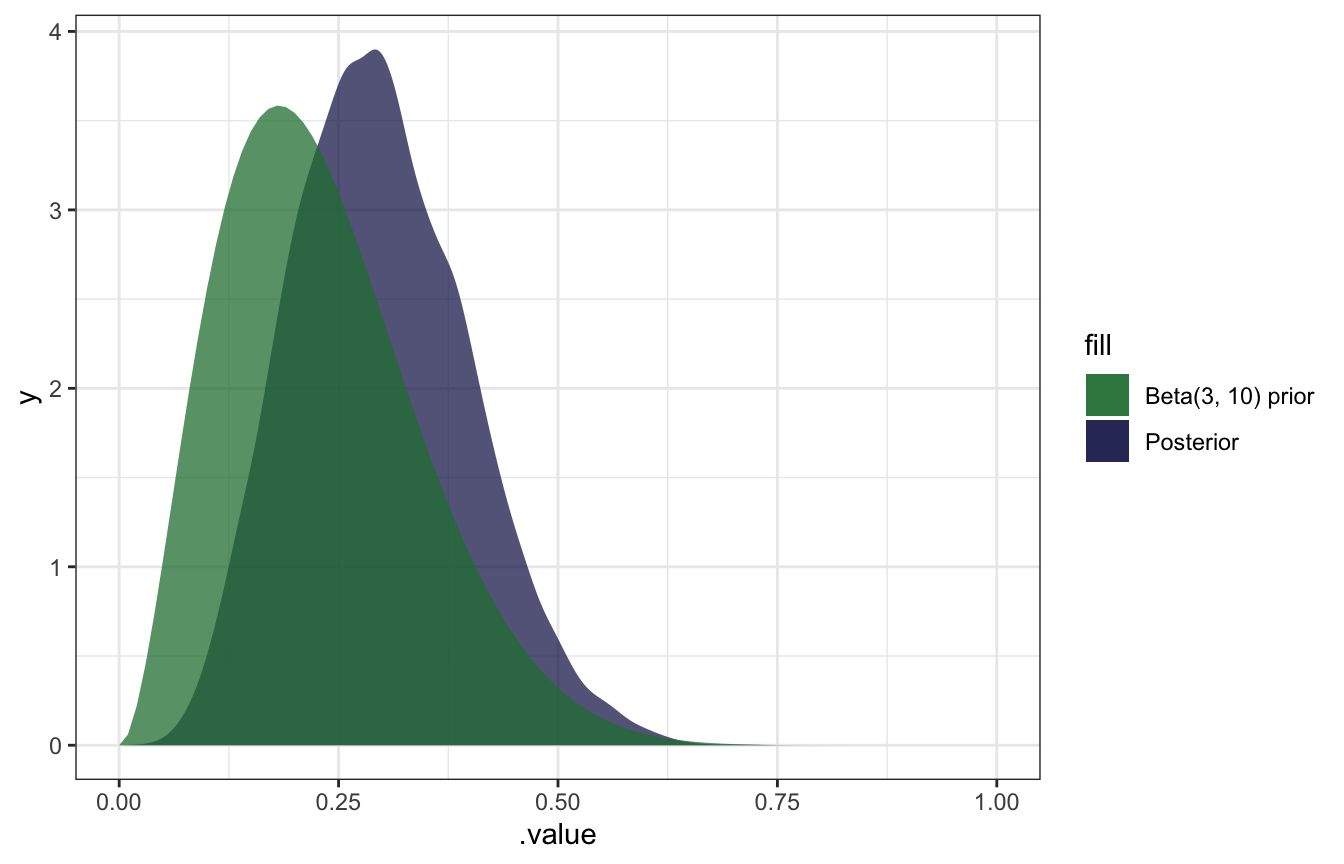pi_stan.stan:

// Things coming in from R
data {
int<lower=0> total_days;  // Possible days open (corresponds to binomial trials)
int<lower=0> days_open;  // Outcome variable
}

// Things to estimate
parameters {
real<lower=0, upper=1> pi;  // Probability the store is open
}

// Models and distributions
model {
// Prior
pi ~ beta(3, 10);

// Likelihood
days_open ~ binomial(total_days, pi);

// Internally, these ~ formulas really look like this:
//   target += beta_lpdf(pi | 3, 10);
//   target += binomial_lpmf(days_open | total_days, pi);
// The ~ notation is a lot nicer and maps onto the notation more directly
}
model_stan <- rstan::sampling(
pi_stan,
data = list(days_open = 3, total_days = 7),
iter = 5000, warmup = 1000, seed = BAYES_SEED, chains = 4
)
model_stan %>%
summarize(across(pi, lst(mean, median)))
## # A tibble: 1 × 2
##   pi_mean pi_median
##     <dbl>     <dbl>
## 1   0.298     0.292
model_stan %>%
ggplot(aes(x = pi)) +
stat_halfeye()
## Warning: Using the size aesthietic with geom_segment was deprecated in ggplot2 3.4.0.
## ℹ Please use the linewidth aesthetic instead.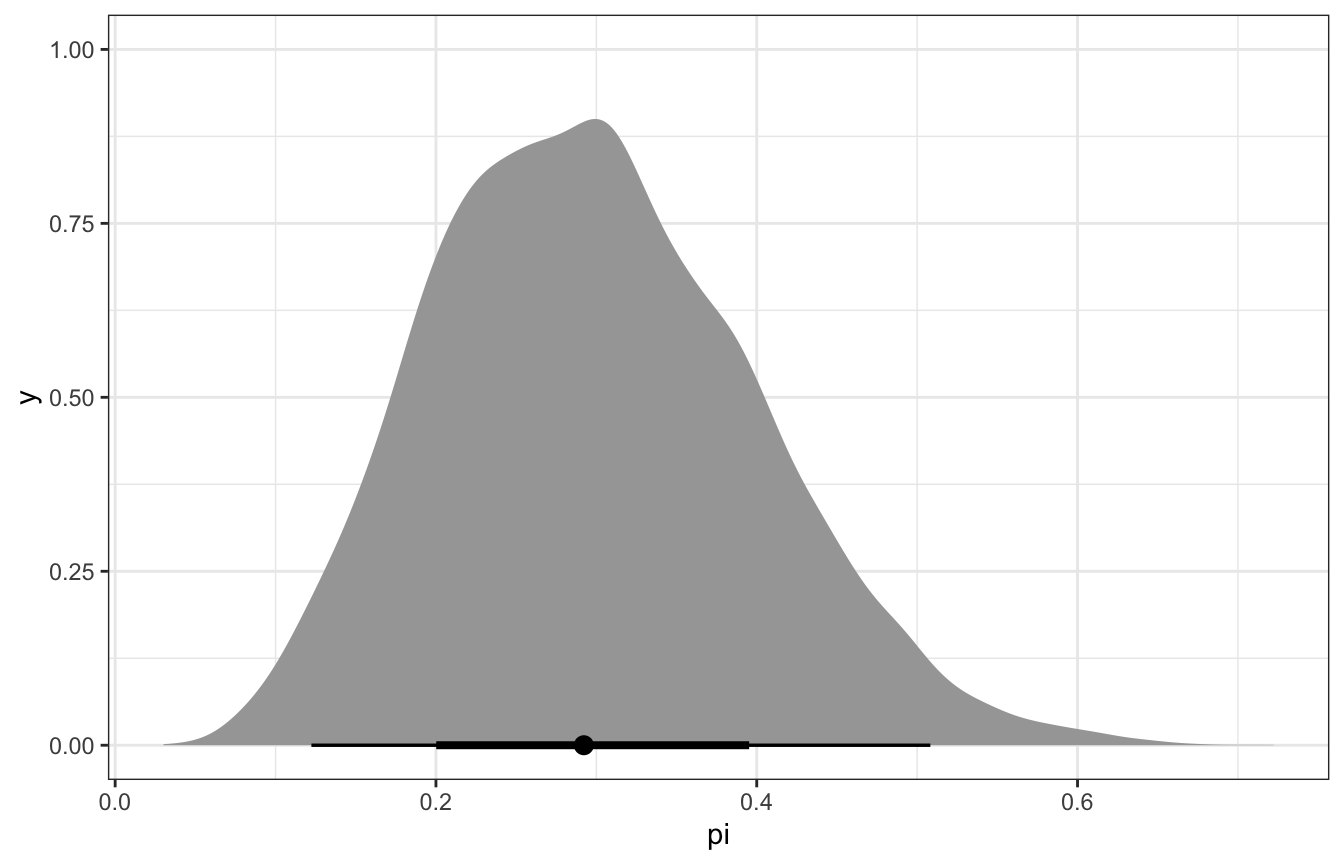From equation 3.10 in Bayes Rules!:

\begin{aligned} \pi \mid (Y = y) &\sim \operatorname{Beta}(\alpha + y, \quad \beta + n - y) \\ \pi \mid (Y = 3) &\sim \operatorname{Beta}(3 + 3, \quad 10 + 7 - 3) \\ &\sim \operatorname{Beta}(6, 14) \end{aligned}

ggplot() +
geom_function(fun = ~dbeta(., 6, 14))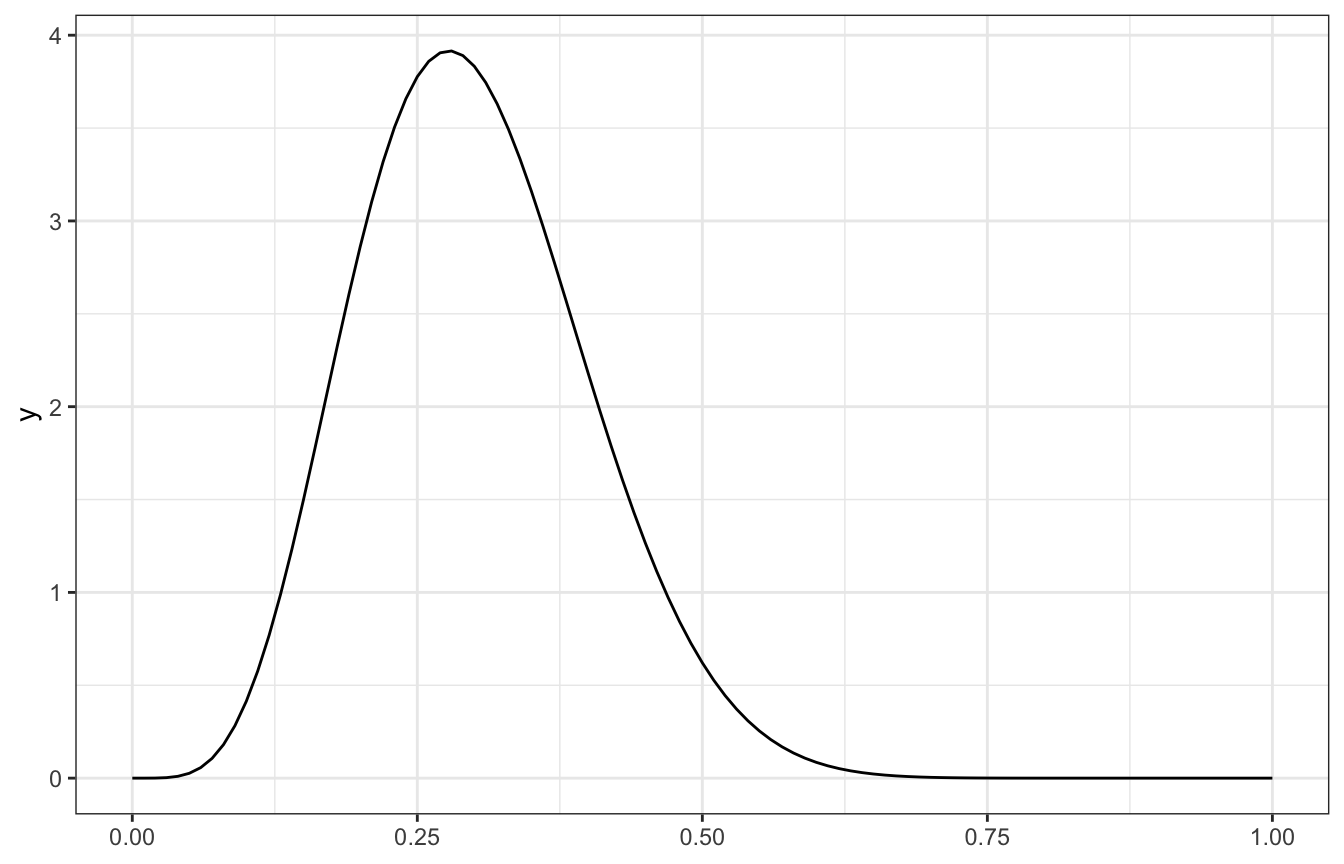And from equation 3.11 in Bayes Rules!:

\begin{aligned} E(\pi \mid Y = y) &= \frac{\alpha + y}{\alpha + \beta + n} \\ E(\pi \mid Y = 3) &= \frac{3 + 3}{3 + 10 + 7} \\ &= \frac{6}{20} = 0.3 \\ \end{aligned}

\begin{aligned} \operatorname{Mode}(\pi \mid Y = y) &= \frac{\alpha + y - 1}{\alpha + \beta + n - 2} \\ \operatorname{Mode}(\pi \mid Y = 3) &= \frac{3 + 3 - 1}{3 + 10 + 7 - 2} \\ &= \frac{5}{18} = 0.27\bar{7} \\ \end{aligned}

\begin{aligned} \operatorname{Var}(\pi \mid Y = y) &= \frac{(\alpha + y)~(\beta + n - y)}{(\alpha + \beta + n)^2~(\alpha + \beta + n + 1)} \\ \operatorname{Var}(\pi \mid Y = 3) &= \frac{(3 + 3)~(10 + 7 - 3)}{(3 + 10 + 7)^2~(3 + 10 + 7 + 1)} \\ &= \frac{6 \times 14}{20^2 \times 21} = \frac{84}{8,400} = 0.01 \\ \operatorname{SD}(\pi \mid Y = 3) &= \sqrt{0.01} = 0.1 \end{aligned}

Verify using summarize_beta_binomial():

summarize_beta_binomial(alpha = 3, beta = 10, y = 3, n = 7)
##       model alpha beta      mean      mode        var        sd
## 1     prior     3   10 0.2307692 0.1818182 0.01267963 0.1126039
## 2 posterior     6   14 0.3000000 0.2777778 0.01000000 0.1000000

#### All four coworkers

(sans raw Stan; brms is fine and great for this anyway)

# Clean this table of coworkers up a bit
priors_clean <- worker_prior_densities %>%
select(coworker, prior, shape1, shape2)

priors_clean %>%
knitr::kable()
coworker prior shape1 shape2
Kimya Beta(1, 2) 1.0 2.0
Fernando Beta(0.5, 1) 0.5 1.0
Ciara Beta(3, 10) 3.0 10.0
Taylor Beta(2, 0.1) 2.0 0.1
br_simulation_pi <- priors_clean %>%
mutate(pi_sim = map2(shape1, shape2, ~{
tibble(pi = rbeta(10000, .x, .y)) %>%
mutate(y = rbinom(10000, size = 7, prob = pi))
}))

br_simulation_pi %>%
unnest(pi_sim) %>%
ggplot(aes(x = pi, y = y)) +
geom_point(aes(color = (y == 3))) +
facet_wrap(vars(coworker))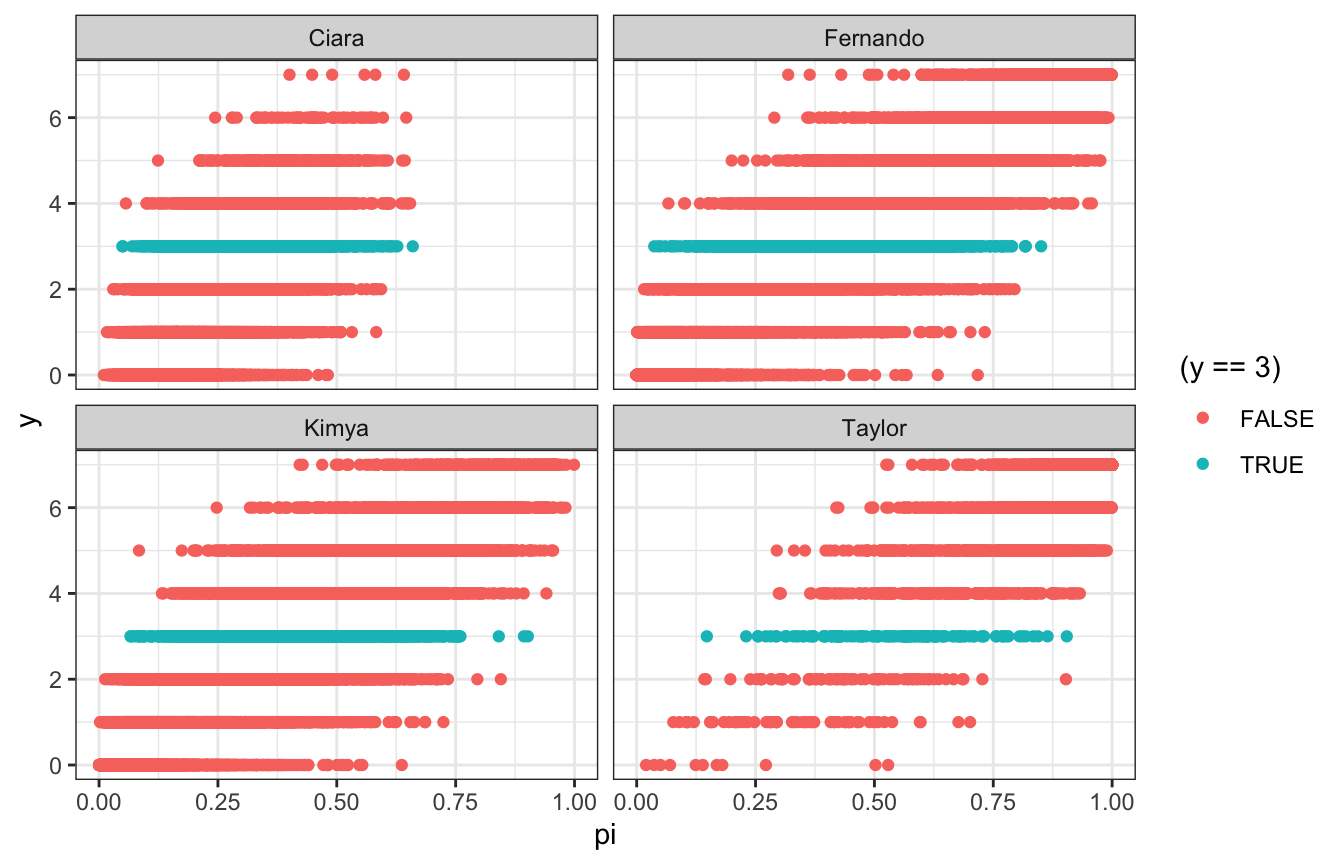br_simulation_pi %>%
unnest(pi_sim) %>%
filter(y == 3) %>%
group_by(coworker) %>%
summarize(across(pi, lst(mean, median)))
## # A tibble: 4 × 3
##   coworker pi_mean pi_median
##   <chr>      <dbl>     <dbl>
## 1 Ciara      0.305     0.297
## 2 Fernando   0.416     0.414
## 3 Kimya      0.400     0.391
## 4 Taylor     0.545     0.564

br_simulation_pi %>%
unnest(pi_sim) %>%
filter(y == 3) %>%
ggplot(aes(x = pi, fill = coworker, color = coworker)) +
geom_density(size = 1, alpha = 0.4) +
scale_fill_manual(values = clrs[c(1, 2, 3, 5)]) +
scale_color_manual(values = clrs[c(1, 2, 3, 5)])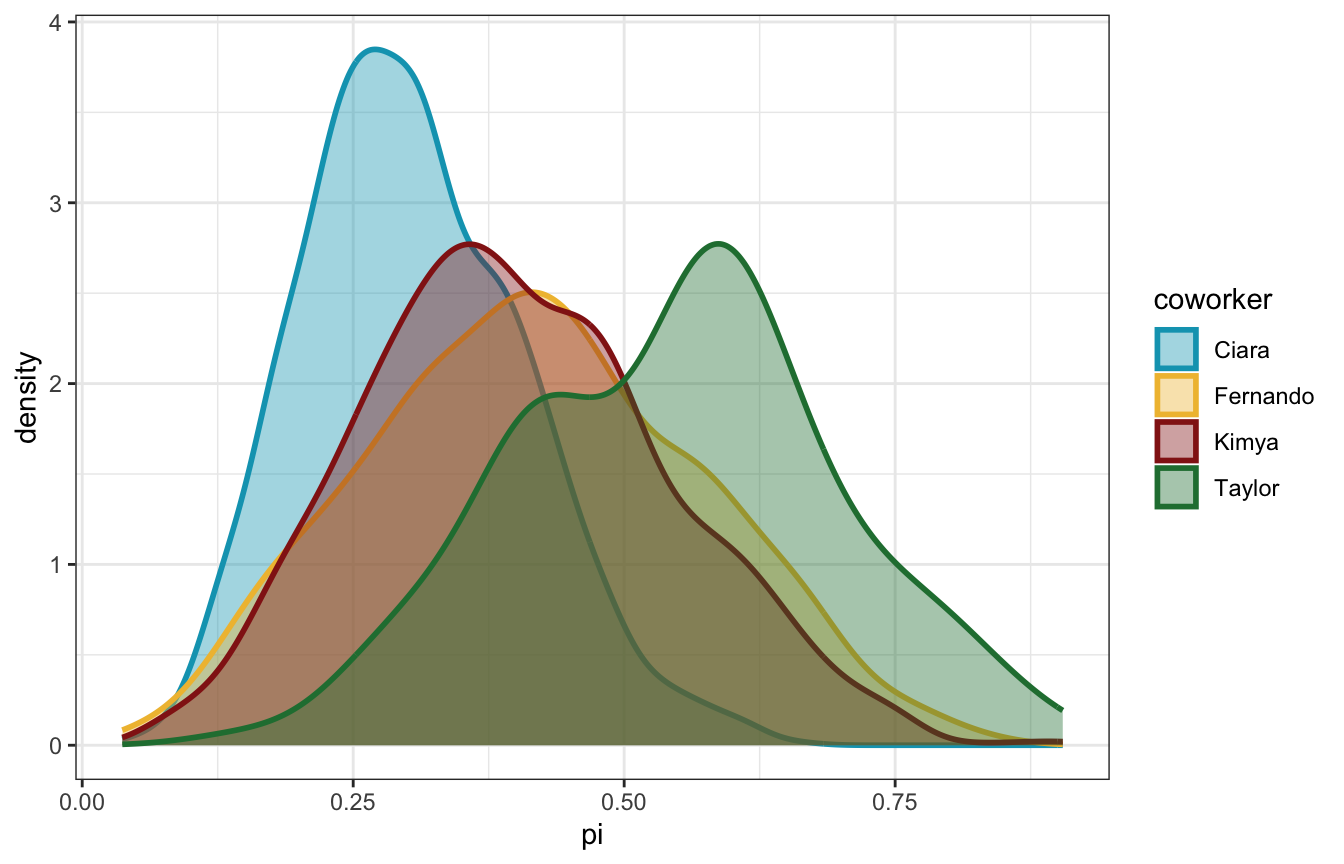Cool cool. Fernando and Kimya’s flatter priors end up leading to posteriors of ≈40%. Taylor’s extreme optimism leads to a posterior mean of 57%! Ciara’s more reasonable range leads to a posterior of 30%.

grid_simulation_pi <- priors_clean %>%
mutate(pi_grid = list(seq(0.01, 0.99, length.out = 1001))) %>%
mutate(prior_beta = pmap(list(pi_grid, shape1, shape2), ~{
dbeta(..1, ..2, ..3)
})) %>%
mutate(likelihood = map(pi_grid, ~{
dbinom(3, size = 7, prob = .x)
})) %>%
mutate(posterior = map2(prior_beta, likelihood, ~{
(.y * .x) / sum(.y * .x)
}))

grid_simulation_samples <- grid_simulation_pi %>%
unnest(c(pi_grid, posterior)) %>%
group_by(coworker) %>%
slice_sample(n = 10000, weight_by = posterior, replace = TRUE)

grid_simulation_samples %>%
group_by(coworker) %>%
summarize(across(pi_grid, lst(mean, median)))
## # A tibble: 4 × 3
##   coworker pi_grid_mean pi_grid_median
##   <chr>           <dbl>          <dbl>
## 1 Ciara           0.300          0.294
## 2 Fernando        0.412          0.405
## 3 Kimya           0.399          0.394
## 4 Taylor          0.550          0.555

grid_simulation_samples %>%
ggplot(aes(x = pi_grid, fill = coworker, color = coworker)) +
geom_density(size = 1, alpha = 0.4) +
scale_fill_manual(values = clrs[c(1, 2, 3, 5)]) +
scale_color_manual(values = clrs[c(1, 2, 3, 5)])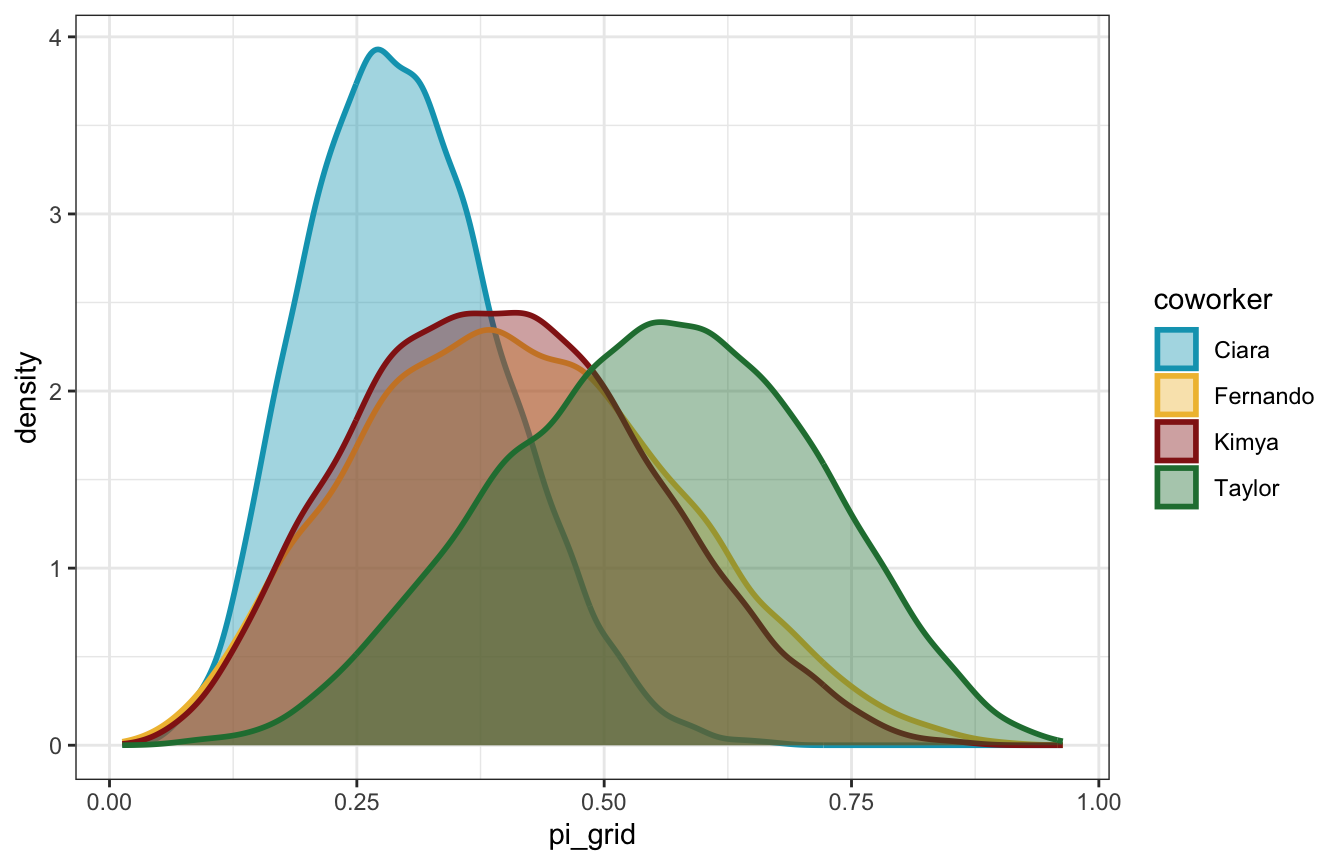brms_pi <- priors_clean %>%
mutate(stan_prior = map2(shape1, shape2, ~{
prior_string(glue::glue("beta({.x}, {.y})"), class = "b", lb = 0, ub = 1)
})) %>%
mutate(model = map(stan_prior, ~{
brm(
bf(days_open | trials(weekdays) ~ 0 + Intercept),
data = list(days_open = 3, weekdays = 7),
prior = .x,
iter = 5000, warmup = 1000, seed = BAYES_SEED,
backend = "rstan", cores = 4
)
}))
## Compiling Stan program...
## Start sampling
## Compiling Stan program...
## Start sampling
## Compiling Stan program...
## Start sampling
## Compiling Stan program...
## Start sampling
brms_pi %>%
mutate(draws = map(model, ~spread_draws(., b_Intercept))) %>%
unnest(draws) %>%
group_by(coworker) %>%
summarize(across(b_Intercept, lst(mean, median, hdci = ~median_hdci(., width = 0.89)))) %>%
unnest(b_Intercept_hdci)
## # A tibble: 4 × 9
##   coworker b_Intercept_mean b_Intercep…¹     y  ymin  ymax .width .point .inte…²
##   <chr>               <dbl>        <dbl> <dbl> <dbl> <dbl>  <dbl> <chr>  <chr>
## 1 Ciara               0.298        0.292 0.292 0.110 0.493   0.95 median hdci
## 2 Fernando            0.411        0.403 0.403 0.125 0.726   0.95 median hdci
## 3 Kimya               0.399        0.392 0.392 0.130 0.693   0.95 median hdci
## 4 Taylor              0.547        0.552 0.552 0.258 0.850   0.95 median hdci
## # … with abbreviated variable names ¹​b_Intercept_median, ²​.interval
brms_pi %>%
mutate(draws = map(model, ~gather_draws(., b_Intercept))) %>%
unnest(draws) %>%
ggplot(aes(x = .value, fill = coworker)) +
stat_halfeye(alpha = 0.4) +
scale_fill_manual(values = clrs[c(1, 2, 3, 5)])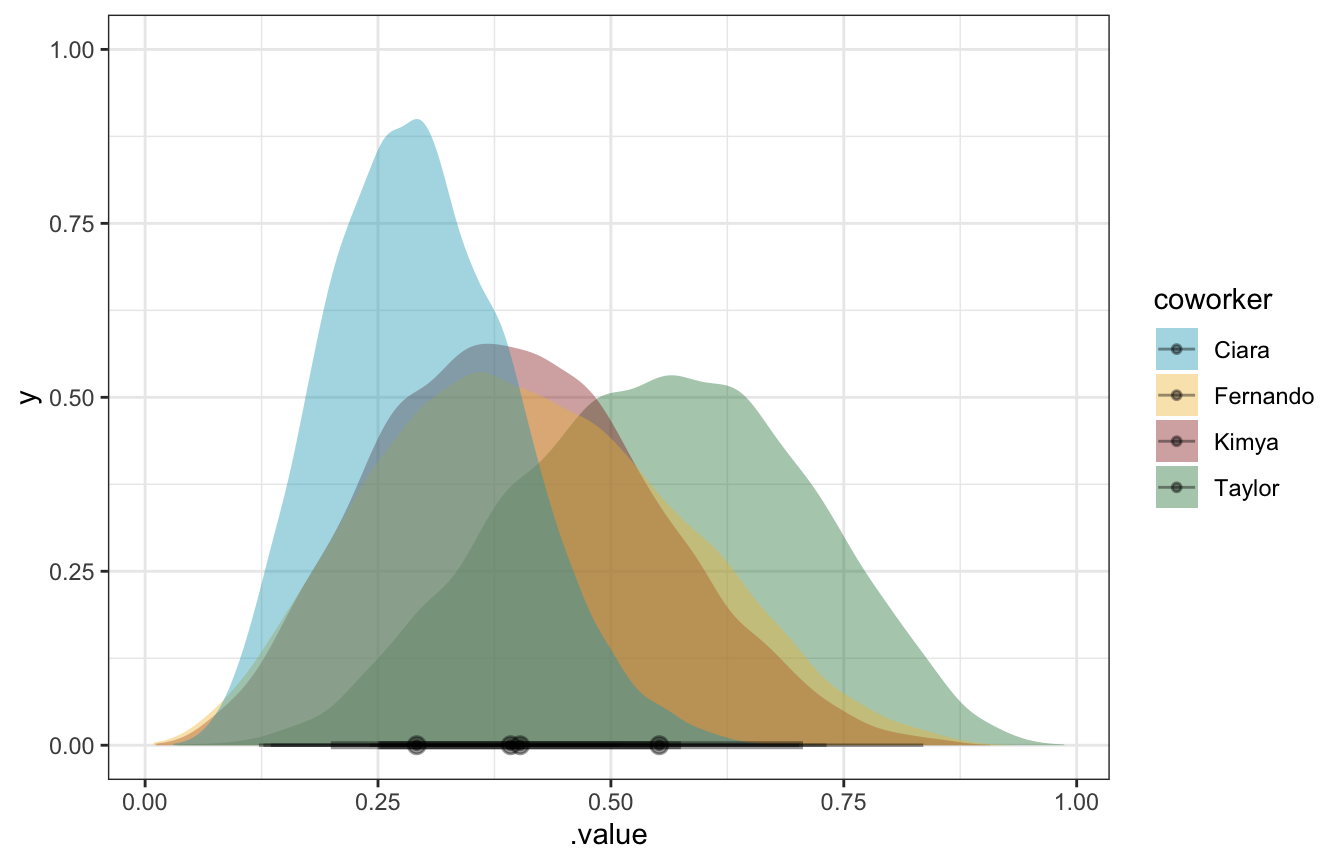lik_y <- 3
lik_n <- 7

posteriors_manual <- priors_clean %>%
mutate(posterior_shape1 = shape1 + lik_y,
posterior_shape2 = shape2 + lik_n - lik_y)

posteriors_manual %>%
mutate(posterior = glue::glue("Beta({posterior_shape1}, {posterior_shape2})")) %>%
select(coworker, prior, posterior) %>%
knitr::kable()
coworker prior posterior
Kimya Beta(1, 2) Beta(4, 6)
Fernando Beta(0.5, 1) Beta(3.5, 5)
Ciara Beta(3, 10) Beta(6, 14)
Taylor Beta(2, 0.1) Beta(5, 4.1)
posteriors_manual_plot <- posteriors_manual %>%
mutate(range = list(seq(0.01, 0.99, length.out = 1001))) %>%
mutate(density = pmap(list(range, posterior_shape1, posterior_shape2), ~dbeta(..1, ..2, ..3)))

posteriors_manual_plot %>%
unnest(c(range, density)) %>%
ggplot(aes(x = range, y = density, fill = coworker, color = coworker)) +
geom_line(size = 1) +
geom_area(position = position_identity(), alpha = 0.4) +
scale_fill_manual(values = clrs[c(1, 2, 3, 5)]) +
scale_color_manual(values = clrs[c(1, 2, 3, 5)])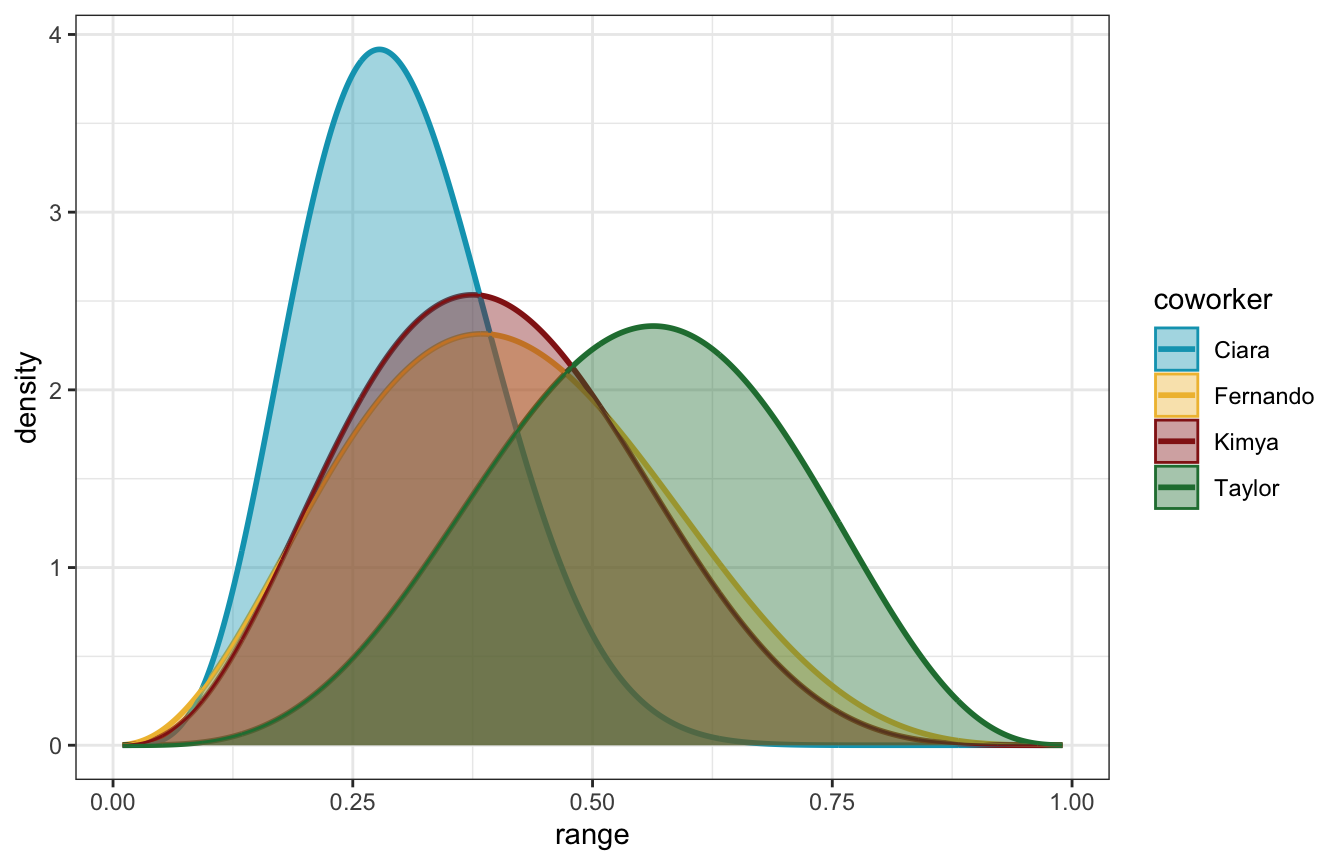posteriors_manual_summary <- posteriors_manual %>%
group_by(coworker) %>%
summarize(summary = map2(shape1, shape2, ~{
summarize_beta_binomial(alpha = .x, beta = .y, y = lik_y, n = lik_n)
})) %>%
unnest(summary)

posteriors_manual_summary %>%
select(-coworker) %>%
kableExtra::kbl(digits = 3) %>%
kableExtra::pack_rows(index = table(posteriors_manual_summary\$coworker)) %>%
kableExtra::kable_styling()
model alpha beta mean mode var sd
Ciara
prior 3.0 10.0 0.231 0.182 0.013 0.113
posterior 6.0 14.0 0.300 0.278 0.010 0.100
Fernando
prior 0.5 1.0 0.333 1.000 0.089 0.298
posterior 3.5 5.0 0.412 0.385 0.025 0.160
Kimya
prior 1.0 2.0 0.333 0.000 0.056 0.236
posterior 4.0 6.0 0.400 0.375 0.022 0.148
Taylor
prior 2.0 0.1 0.952 1.000 0.015 0.121
posterior 5.0 4.1 0.549 0.563 0.025 0.157